Author: Oscar Cronquist Article last updated on March 22, 2023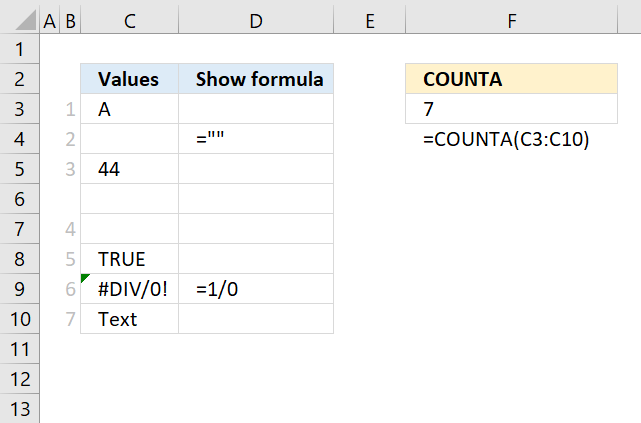The COUNTA function counts the non-empty or non-blank cells in a cell reference.

## 1. COUNTA Function Syntax

COUNTA(value1, [value2], ...)

## 2. COUNTA Function Arguments

 value1 Required. A cell reference to a range for which you want to count not empty values. [value2] Optional. Up to 254 additional arguments like the one above.

The COUNTA function counts errors as not empty.

## 3. COUNTA Function exampleFormula in cell F3:

=COUNTA(C3:C10)

The picture above demonstrates the COUNTA function entered in cell F3. The evaluated range is C3:C10, two of the cells contain formulas, and three cells seem to be empty but only one is in fact empty.

Cell C4 has a formula that returns a blank, note that the COUNTA function considers this cell not empty. Cell C7 has a space character and is therefore counted. Only cell C6 is empty.

## 4. Count non-empty values in an array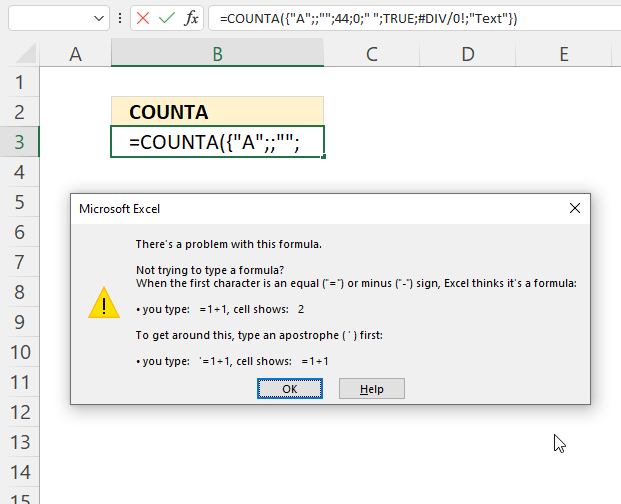The COUNTA function returns an error dialog box if at least one of the containers is empty.

Formula in cell B3:

=COUNTA({"A";;"";44;0;" ";TRUE;#DIV/0!;"Text"})

The formula above does not work, however, the formula below works.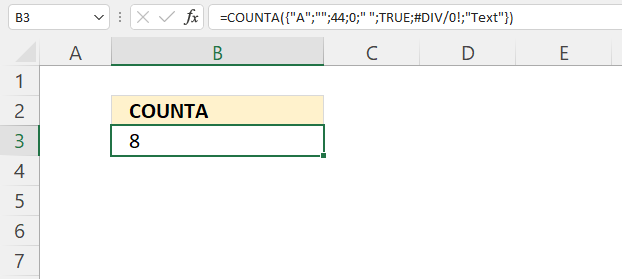The COUNTA function works if all containers in the array are non-empty.

Formula in cell B3:

=COUNTA({"A";"";44;0;" ";TRUE;#DIV/0!;"Text"})

An empty container like this "" is not considered empty which is surprising. The following formula counts non empty values in a hardcoded array.

=SUMPRODUCT((IFERROR({"A";"";44;0;" ";TRUE;#DIV/0!;"Text"},"A")<>"")*1)

### Explaining formula

#### Step 1 - Replace errors with a text value

The IFERROR function catches most errors in Excel formulas.
IFERROR(valuevalue_if_error)

IFERROR({"A";"";44;0;" ";TRUE;#DIV/0!;"Text"},"A")

returns

{"A"; ""; 44; 0; " "; TRUE; "A"; "Text"}

#### Step 2 - Check if not empty

The less than and the larger than characters combined lets you test if a value is not equal to another value. The result is either TRUE or FALSE.

(IFERROR({"A";"";44;0;" ";TRUE;#DIV/0!;"Text"},"A")<>""

becomes

{"A"; ""; 44; 0; " "; TRUE; "A"; "Text"}<>""

and returns

{TRUE; FALSE; TRUE; TRUE; TRUE; TRUE; TRUE; TRUE}.

#### Step 3 - Convert boolean values to the numerical equivalents

This step is need in order to calculate a sum, the SUMPRODUCT function in the next step can't work with boolean values, however, their numerical equivalents work fine.

(IFERROR({"A";"";44;0;" ";TRUE;#DIV/0!;"Text"},"A")<>"")*1

becomes

{TRUE; FALSE; TRUE; TRUE; TRUE; TRUE; TRUE; TRUE}*1

returns

{1; 0; 1; 1; 1; 1; 1; 1}.

#### Step 4 - Calculate a total

The SUMPRODUCT function calculates the product of corresponding values and then returns the sum of each multiplication.
SUMPRODUCT(array1, [array2], ...)

SUMPRODUCT((IFERROR({"A";"";44;0;" ";TRUE;#DIV/0!;"Text"},"A")<>"")*1)

becomes

SUMPRODUCT({1; 0; 1; 1; 1; 1; 1; 1})

and returns 8.

## 5. Count non-empty values based on a condition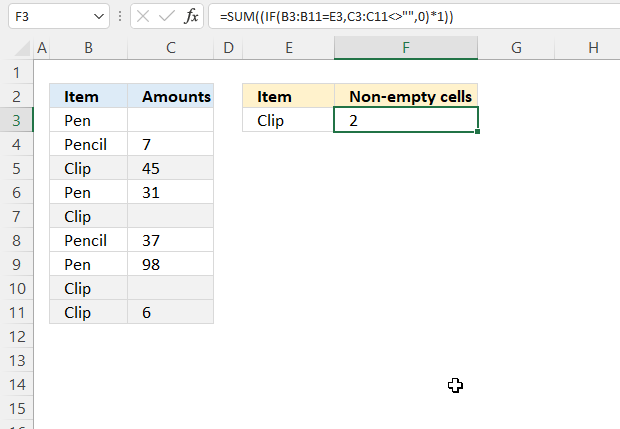This example demonstrates how to count nonblank cells based on a condition applied to an adjacent column. The image above shows the condition in cell E3, cell range B3:B11 contains the values and the corresponding values in cell range C3:C11 contain numbers and some blank cells.

The formula in cell F3 matches the condition to B3:B11 and the corresponding matching cells are C5, C10 and C11. Cells C5 and C11 contain numbers, however, cell C10 is blank. The formula returns 2, there are two nonempty cells based on the given condition.

Formula in cell F3:

=SUM(IF(B3:B11=E3,C3:C11<>"",0)*1)

### Explaining formula

#### Step 1 - Logical expression

The equal sign lets you compare value to value, it can also be used to compare a single value to multiple different values. The result is a boolean value, it is either TRUE or FALSE.

B3:B11=E3

becomes

{"Pen"; "Pencil"; "Clip"; "Pen"; "Clip"; "Pencil"; "Pen"; "Clip"; "Clip"}="Clip"

and returns

{FALSE; FALSE; TRUE; FALSE; TRUE; FALSE; FALSE; TRUE; TRUE}.

#### Step 2 - Check if not empty (non-empty)

The less than sign and the larger than sign combined lets you check if a value is NOT equal to another value. We are comparing nothing in this example to multiple cells, there is nothing between the double quotes "". The result is a boolean value, it is either TRUE or FALSE or in this case an array containing boolean values.

C3:C11<>""

becomes

{""; 7; 45; 31; ""; 37; 98; ""; 6}<>""

and returns

{FALSE; TRUE; TRUE; TRUE; FALSE; TRUE; TRUE; FALSE; TRUE}

#### Step 3 - Evaluate IF function

The IF function returns one value if the logical test is TRUE and another value if the logical test is FALSE.
IF(logical_test, [value_if_true], [value_if_false])

IF(B3:B11=E3,C3:C11<>"",0)

becomes

IF({FALSE; FALSE; TRUE; FALSE; TRUE; FALSE; FALSE; TRUE; TRUE},C3:C11<>"",0)

becomes

IF({FALSE; FALSE; TRUE; FALSE; TRUE; FALSE; FALSE; TRUE; TRUE},{FALSE; TRUE; TRUE; TRUE; FALSE; TRUE; TRUE; FALSE; TRUE},0)

and returns

{0; 0; TRUE; 0; FALSE; 0; 0; FALSE; TRUE}

#### Step 4 - Convert boolean values

The SUM function can't calculate a total using boolean values, we need to convert the boolean values to their numerical equivalents.

TRUE - 1
FALSE - 0 (zero)

The asterisk lets you multiply numbers and boolean values in an Excel formula.

IF(B3:B11=E3,C3:C11<>"",0)*1

becomes

{0; 0; TRUE; 0; FALSE; 0; 0; FALSE; TRUE}*1

and returns

{0; 0; 1; 0; 0; 0; 0; 0; 1}. All values in the array are now either 1 or 0 (zero).

#### Step 5 - Calculate a total

The SUM function adds values and returns a total.
SUM(number1, [number2], ...)

SUM(IF(B3:B11=E3,C3:C11<>"",0)*1)

becomes

SUM({0; 0; 1; 0; 0; 0; 0; 0; 1})

and returns 2.

## 6. Count non-empty values based on a list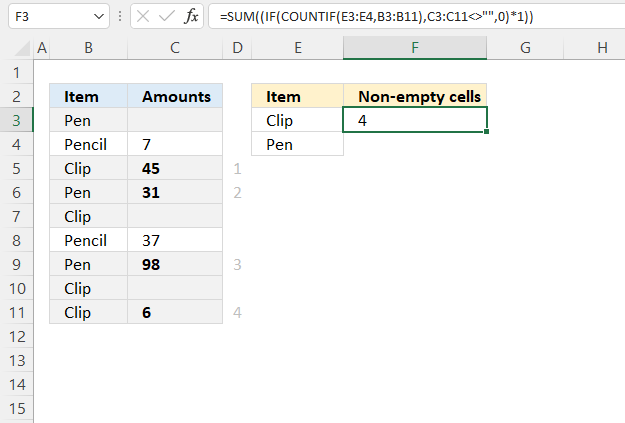This example shows how to use multiple conditions compared to the same cell range B3:B11. The formula counts non-empty cells based on the given criteria.

The image above shows the criteria in cell E3 and E4, the matching cells in cell range B3:B11 are B3, B5, B6, B7, B9, B10 and B11. The corresponding cells on the same row in column C have four non-empty cells. They are C5, C6, C9, and C11.

Formula in cell F3:

=SUM(IF(COUNTIF(E3:E4, B3:B11), C3:C11<>"", 0)*1)

### Explaining formula

#### Step 1 - Which values equal any item in the list

The COUNTIF function counts the number of cells that meet a given condition.
COUNTIF(rangecriteria)

COUNTIF(E3:E4, B3:B11)

becomes

COUNTIF({"Clip"; "Pen"}, {"Pen"; "Pencil"; "Clip"; "Pen"; "Clip"; "Pencil"; "Pen"; "Clip"; "Clip"})

and returns

{1; 0; 1; 1; 1; 0; 1; 1; 1}.

#### Step 2 - Check if not empty (non-empty)

The less than sign and the larger than sign combined lets you check if a value is NOT equal to another value. This example checks if the values are empty.

C3:C11<>""

becomes

{""; 7; 45; 31; ""; 37; 98; ""; 6}<>""

and returns

{FALSE; TRUE; TRUE; TRUE; FALSE; TRUE; TRUE; FALSE; TRUE}.

#### Step 3 - Evaluate IF function

The IF function returns one value if the logical test is TRUE and another value if the logical test is FALSE.
IF(logical_test, [value_if_true], [value_if_false])

IF(COUNTIF(E3:E4, B3:B11), C3:C11<>"", 0)

becomes

IF({1; 0; 1; 1; 1; 0; 1; 1; 1}, {FALSE; TRUE; TRUE; TRUE; FALSE; TRUE; TRUE; FALSE; TRUE}, 0)

and returns

{FALSE; 0; TRUE; TRUE; FALSE; 0; TRUE; FALSE; TRUE}.

#### Step 4 - Convert boolean values

The SUM function can't calculate a total using boolean values, we need to convert the boolean values to their numerical equivalents.

TRUE - 1
FALSE - 0 (zero)

The asterisk lets you multiply numbers and boolean values in an Excel formula.

IF(B3:B11=E3,C3:C11<>"",0)*1

becomes

{FALSE; 0; TRUE; TRUE; FALSE; 0; TRUE; FALSE; TRUE}*1

and returns

{0; 0; 1; 1; 0; 0; 1; 0; 1}.

#### Step 5 - Calculate a total

The SUM function adds values and returns a total.
SUM(number1, [number2], ...)

SUM(IF(B3:B11=E3,C3:C11<>"",0)*1)

becomes

SUM({0; 0; 1; 1; 0; 0; 1; 0; 1})

and returns 4.

## 7. Count non-empty values in a string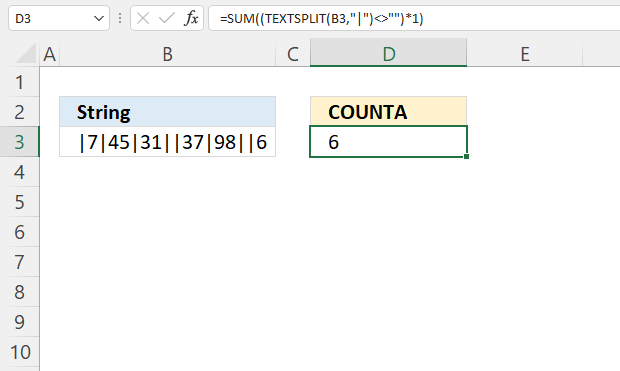This example demonstrates how to count non-blank values in a string of delimited values. The image above shows a string in cell B3 containing the delimiting value ; (semicolon).

Excel 365 dynamic array formula in cell C3:

=SUM((TEXTSPLIT(C3,"|")<>"")*1)

### Explaining formula

#### Step 1 - Split values

The TEXTSPLIT function lets you split a string into an array across columns and rows based on delimiting characters.
TEXTSPLIT(Input_Textcol_delimiter, [row_delimiter], [Ignore_Empty])

TEXTSPLIT(C3,";")

becomes

TEXTSPLIT("|7|45|31||37|98||6","|")

and returns

{"", "7", "45", "31", "", "37", "98", "", "6"}.

#### Step 2 - Check if values in array are not empty

The less than sign and the larger than sign combined lets you check if a value is NOT equal to another value. This example checks if the values are empty.

TEXTSPLIT(C3,";")<>""

becomes

{"", "7", "45", "31", "", "37", "98", "", "6"}<>""

and returns

{FALSE, TRUE, TRUE, TRUE, FALSE, TRUE, TRUE, FALSE, TRUE}.

#### Step 3 - Convert boolean values

The SUM function can't calculate a total using boolean values, we need to convert the boolean values to their numerical equivalents.

TRUE - 1
FALSE - 0 (zero)

The asterisk lets you multiply numbers and boolean values in an Excel formula.

(TEXTSPLIT(C3,";")<>"")*1

becomes

{FALSE, TRUE, TRUE, TRUE, FALSE, TRUE, TRUE, FALSE, TRUE}*1

and returns

{0, 1, 1, 1, 0, 1, 1, 0, 1}

#### Step 4 - Add numbers and return a total

The SUM function adds values and returns a total.
SUM(number1, [number2], ...)

SUM((TEXTSPLIT(C3,";")<>"")*1)

becomes SUM({0, 1, 1, 1, 0, 1, 1, 0, 1})

and returns 6.

## 8. Count non-empty cells in multiple cell ranges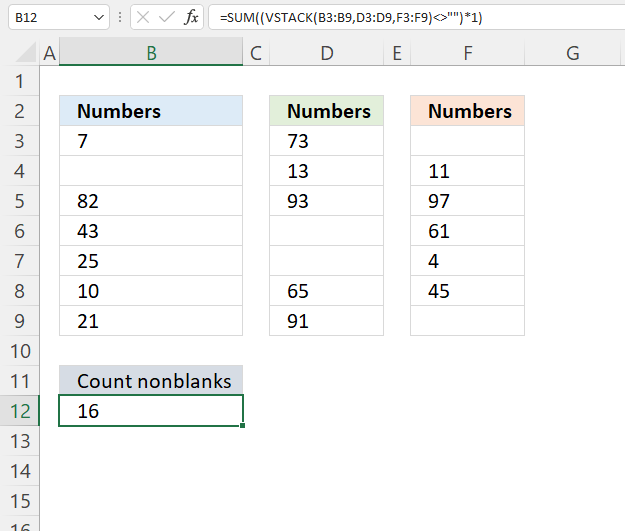This example demonstrates a formula that counts non-empty cells in multiple cell ranges. The new Excel 365 array functions make this easy and straightforward.

The image above shows three nonadjacent cell ranges, they don't need to be the same size vertically although they are in this example. The cell ranges contain values and some random blank cells, the dynamic array formula in cell B12 counts nonblank cells in all three cell ranges combined.

Formula in cell B12:

=SUM((VSTACK(B3:B9, D3:D9, F3:F9)<>"")*1)

### Explaining formula

#### Step 1 - Join arrays

The VSTACK function combines cell ranges or arrays, it joins data to the first blank cell at the bottom of a cell range or array.
VSTACK(array1,[array2],...)

VSTACK(B3:B9, D3:D9, F3:F9)

becomes

VSTACK({7;"";82;43;25;10;21},{73;13;93;"";"";65;91},{"";11;97;61;4;45;""})

and returns

{7; ""; 82; 43; 25; 1""; 21; 73; 13; 93; ""; ""; 65; 91; ""; 11; 97; 61; 4; 45; ""}.

#### Step 2 - Check if not empty

The less than sign and the larger than sign combined lets you check if a value is NOT equal to another value. This example checks if the values are empty.

VSTACK(B3:B9,D3:D9,F3:F9)<>""

becomes

{7; ""; 82; 43; 25; 1""; 21; 73; 13; 93; ""; ""; 65; 91; ""; 11; 97; 61; 4; 45; ""}<>""

and returns

{TRUE; FALSE; TRUE; ... ; FALSE}.

#### Step 3 - Convert boolean values to numbers

The SUM function can't calculate a total using boolean values, we need to convert the boolean values to their numerical equivalents.

TRUE - 1
FALSE - 0 (zero)

The asterisk lets you multiply numbers and boolean values in an Excel formula.

(VSTACK(B3:B9,D3:D9,F3:F9)<>"")*1

becomes

{TRUE; FALSE; TRUE; ... ; FALSE}*1

and returns

{1; 0; 1; 1; 1; 1; 1; 1; 1; 1; 0; 0; 1; 1; 0; 1; 1; 1; 1; 1; 0}.

#### Step 4 - Calculate a total

SUM((VSTACK(B3:B9,D3:D9,F3:F9)<>"")*1)

becomes

SUM({1; 0; 1; 1; 1; 1; 1; 1; 1; 1; 0; 0; 1; 1; 0; 1; 1; 1; 1; 1; 0})

and returns 16.

## 9. Count non-empty cells per row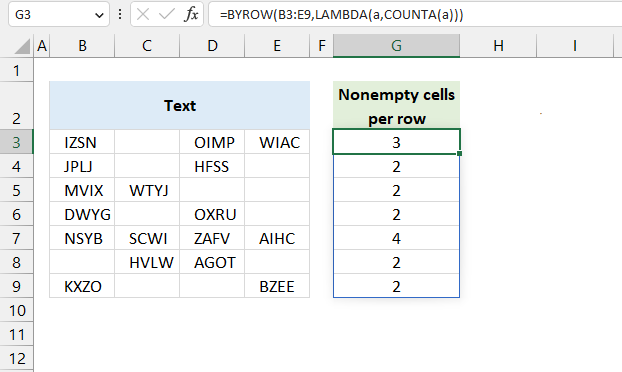The image above shows an Excel 365 dynamic array formula that returns an array of numbers. It counts the number of nonempty cells per row, and the position in the array corresponds to the row in cell range B3:E9.

Excel 365 formula in cell G3:

=BYROW(B3:E9,LAMBDA(a,COUNTA(a)))

### Explaining formula

#### Step 1 - Count nonempty cells

The COUNTA function counts the non-empty or non-blank cells in a cell range.

Function syntax: COUNTA(value1, [value2], ...)

COUNTA(a)

#### Step 2 - Build the LAMBDA function

The LAMBDA function build custom functions without VBA, macros or javascript.

Function syntax: LAMBDA([parameter1, parameter2, …,] calculation)

LAMBDA(a,COUNTA(a))

#### Step 3 - Count nonempty cells per row

The BYROW function puts values from an array into a LAMBDA function row-wise.

Function syntax: BYROW(array, lambda(array, calculation))

BYROW(B3:E9,LAMBDA(a,COUNTA(a)))

## 10. Sort rows based on the number of non-empty cells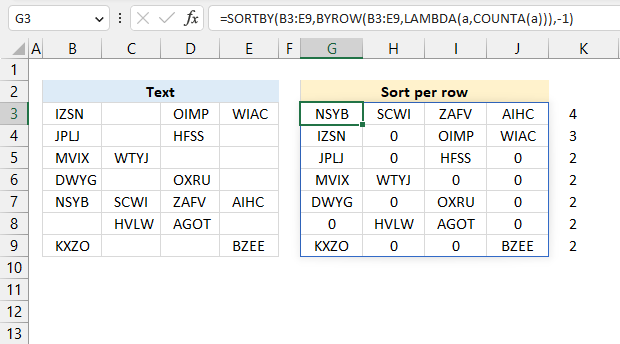Excel 365 dynamic array formula in cell G3:

=SORTBY(B3:E9,BYROW(B3:E9,LAMBDA(a,COUNTA(a))),-1)

### Explaining formula

This formula works just like the example in section 9, but it also sorts rows based on the count of nonempty cells.

The SORTBY function sorts a cell range or array based on values in a corresponding range or array.

Function syntax: SORTBY(array, by_array1, [sort_order1], [by_array2, sort_order2],…)

## 11. Count non-empty cells per column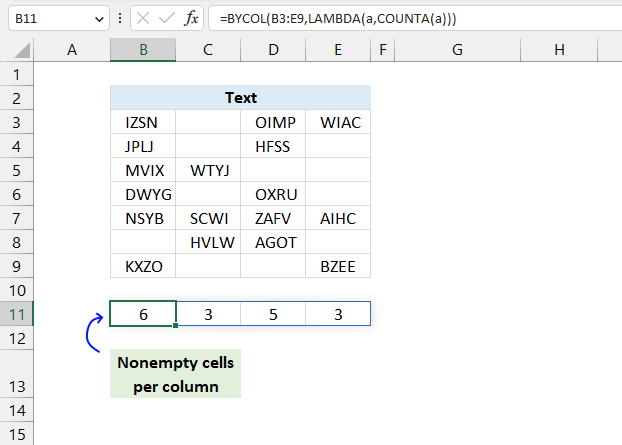The image above shows an Excel 365 dynamic array formula that returns an array of numbers. It counts the number of nonempty cells per column, and the position in the array corresponds to the column in cell range B3:E9.

Excel 365 formula in cell B11:

=BYCOL(B3:E9,LAMBDA(a,COUNTA(a)))

### Explaining formula

#### Step 1 - Count nonempty cells

The COUNTA function counts the non-empty or non-blank cells in a cell range.

Function syntax: COUNTA(value1, [value2], ...)

COUNTA(a)

#### Step 2 - Build the LAMBDA function

The LAMBDA function build custom functions without VBA, macros or javascript.

Function syntax: LAMBDA([parameter1, parameter2, …,] calculation)

LAMBDA(a,COUNTA(a))

#### Step 3 - Count nonempty cells per column

The BYCOL function passes all values in a column based on an array to a LAMBDA function, the LAMBDA function calculates new values based on a formula you specify. It then continues with the next column until all columns in the array have been processed.

Function syntax: BYCOL(array, lambda(array, calculation))

BYCOL(B3:E9,LAMBDA(a,COUNTA(a)))

## 12. Sort columns based on the number of non-empty cells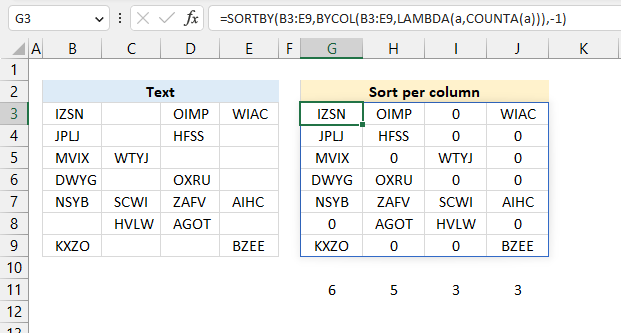Excel 365 dynamic array formula in cell G3:

=SORTBY(B3:E9,BYCOL(B3:E9,LAMBDA(a,COUNTA(a))),-1)

### Explaining formula

This formula works just like the example in section 11, however, it also sorts columns in cell range B3:E9 based on the count of nonempty cells.

The image above shows 6 in cell G11, and there are 6 nonempty cells in G3:G9. The next cell H11 displays 5, there are five nonempty cells in H3:H9.

The SORTBY function sorts a cell range or array based on values in a corresponding range or array.

Function syntax: SORTBY(array, by_array1, [sort_order1], [by_array2, sort_order2],…)